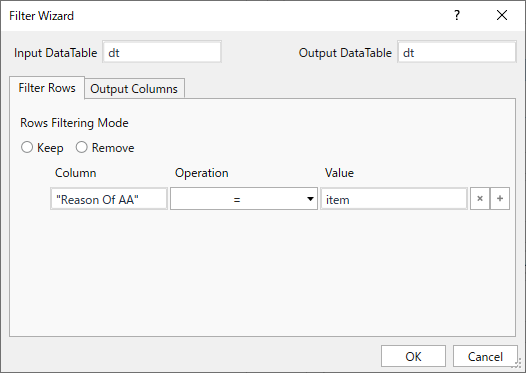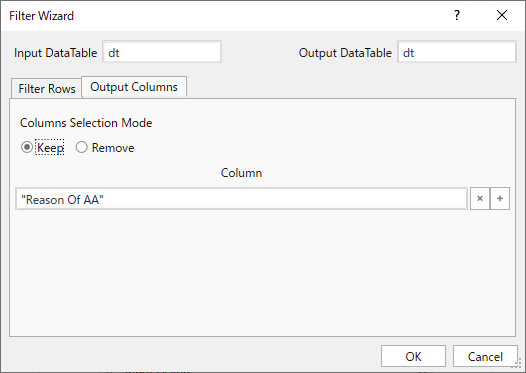# How to edit linq query?

Now Linq query as below.

dt.AsEnumerable.Where(function(r) r(“Reason Of AA”).ToString()=item).CopyToDataTable()

Output show all table that column name AA no = item

It I want output = datatable column name AA no only.

Hi,

Can you try Filter DataTable activity as the following? This can filter specific row and column at once.Regards,

1 Like

`dt.AsEnumerable.Select(function (r) r("Reason Of AA")).ToArray`

@kumar.varun2 What type in variable ?

Array of string

`dt.AsEnumerable.Select(function (r) r("Reason Of AA").ToString).ToArray`

If you want the output in a data table then, build a data table(`outDT`) using Build Data Table activity with one column of type string and use the following LINQ.

`(from r in dt select outDT.Rows.Add(r("Reason Of AA").ToString)).CopyToDataTable`

Hi,

The following will also work, without pre-build datatable, FYI.

``````dt.AsEnumerable.Where(function(r) r("Reason Of AA").ToString()=item).CopyToDataTable().DefaultView.ToTable("NewTable",False,{"Reason Of AA"})
``````

Regards,

3 Likes

Perfect Solution!

1 Like

@Yoichi I have more question.

If I want header in output.
How to edit query?